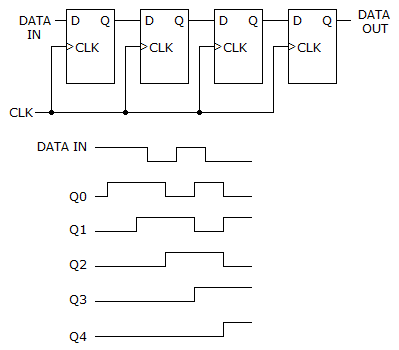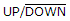# Digital Electronics - Counters

### Exercise :: Counters - General Questions

67.

Which of the following statements are true?

 A. Asynchronous events do not occur at the same time. B. Asynchronous events are controlled by a clock. C. Synchronous events do not need a clock to control them. D. Only asynchronous events need a control clock.

Explanation:

No answer description available for this question. Let us discuss.

68.

Which segments (by letter) of a seven-segment display need to be active in order to display a digit 6?

 A. b, c, d, e, f, and g B. a, c, d, e, f, and g C. a, b, c, d, and f D. b, c, d, e, and f

Explanation:

No answer description available for this question. Let us discuss.

69.

Which of the following groups of logic devices would be the minimum required for a MOD-64 synchronous counter?

 A. Five flip-flops, three AND gates B. Seven flip-flops, five AND gates C. Four flip-flops, ten AND gates D. Six flip-flops, four AND gates

Explanation:

No answer description available for this question. Let us discuss.

70.

The circuit given below fails to produce data output. The individual flip-flops are checked with a logic probe and pulser, and each checks OK. What could be causing the problem?A. The data output line may be grounded. B. One of the clock input lines may be open. C. One of the interconnect lines between two stages may have a solder bridge to ground. D. One of the flip-flops may have a solder bridge between its input and Vcc.

Explanation:

No answer description available for this question. Let us discuss.

71.

A 22-MHz clock signal is put into a MOD-16 counter. What is the frequency of the Q output of each stage of the counter?

 A. Q1 = 22 MHz, Q2 = 11 MHz, Q3 = 5.5 MHz, Q4 = 2.75 MHz B. Q1 = 11 MHz, Q2 = 5.5 MHz, Q3 = 2.75 MHz, Q4 = 1.375 MHz C. Q1 = 11 MHz, Q2 = 11 MHz, Q3 = 11 MHz, Q4 = 11 MHz D. Q1 = 22 MHz, Q2 = 22 MHz, Q3 = 22 MHz, Q4 = 22 MHz

Explanation:

No answer description available for this question. Let us discuss.

72.

The designationmeans that the ________.

 A. up count is active-HIGH, the down count is active-LOW B. up count is active-LOW, the down count is active-HIGH C. up and down counts are both active-LOW D. up and down counts are both active-HIGH